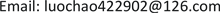﻿ 一类带有饱和发生率和Logistic增长的随机病毒感染模型的灭绝性及平稳分布 Extinction and Stationary Distribution of a Classic Stochastic Viral Infection Model with Saturation Rate and Logistic Growth

Vol.07 No.05(2018), Article ID:25078,8 pages
10.12677/AAM.2018.75072

Extinction and Stationary Distribution of a Classic Stochastic Viral Infection Model with Saturation Rate and Logistic Growth

Chao Luo, Xiaodan Zhang

School of Mathematics and Physics, University of Science and Technology Beijing, BeijingReceived: May 1st, 2018; accepted: May 17th, 2018; published: May 25th, 2018ABSTRACT

In this paper, a classic stochastic viral infection model with saturation rate and logistic growth is researched. To be start, when ${R}_{0}<1$ , the boundeness of uninfected cells and the extinction of infected cells are discussed. Then, when ${R}_{0}>1$ , some available Lyapunov functions are constricted and some sufficient conditions are established for stationary distribution. The theory verifies that infected cells keep persistence in vivo.

Keywords:Saturation Rate, Logistic Growth, Stationary Distribution1. 引言

$\left\{\begin{array}{l}\frac{\text{d}x}{\text{d}t}=s-dx-\beta xy\\ \frac{\text{d}y}{\text{d}t}=\beta xy-\mu y\end{array}$

$\left\{\begin{array}{l}\frac{\text{d}x}{\text{d}t}=s+rx\left(1-\frac{x}{K}\right)-dx-\frac{kxy}{x+y}+{\sigma }_{1}x\frac{\text{d}{B}_{1}\left(t\right)}{\text{d}t}\\ \frac{\text{d}y}{\text{d}t}=\frac{kxy}{x+y}-\left(a+\mu \right)y+{\sigma }_{2}y\frac{\text{d}{B}_{2}\left(t\right)}{\text{d}t}\end{array}$ (1.1)

2. 随机微分方程的基本理论 

$\text{d}X\left(t\right)=f\left(X\left(t\right),t\right)\text{d}t+g\left(X\left(t\right),t\right)\text{d}B\left(t\right)$

$L=\frac{\partial }{\partial t}+\sum _{i=1}^{n}{f}_{i}\left(X,t\right)\frac{\partial }{\partial {x}_{i}}+\frac{1}{2}\sum _{i,j=1}^{n}{\left[{g}^{\text{T}}\left({x}_{i},t\right)g\left({x}_{j},t\right)\right]}_{ij}\frac{{\partial }^{2}}{\partial {x}_{i}\partial {x}_{j}}$

$LV\left(X,t\right)={V}_{t}\left(X,t\right)+{V}_{X}\left(X,t\right)f\left(X,t\right)+\frac{1}{2}trace\left[{g}^{\text{T}}\left(X,t\right){V}_{XX}\left(X,t\right)g\left(X,t\right)\right]$ ,

$\text{d}V\left(x\left(t\right),t\right)=LV\left(x\left(t\right),t\right)\text{d}t+{V}_{X}\left(x\left(t\right),t\right)g\left(x\left(t\right),t\right)\text{d}B\left(t\right)$ .

3. 模型(1)全局正解的存在唯一性和感染细胞的存在与灭绝性

$\underset{t\to \infty }{\mathrm{lim}}\frac{1}{t}{\int }_{0}^{t}{\left(x\left(s\right)-\frac{K\left(r-d\right)}{2r}\right)}^{2}\text{d}s\le \frac{sK}{r}+\frac{{K}^{2}{\left(r-d\right)}^{2}}{4{r}^{2}}$

$\underset{t\to \infty }{\mathrm{lim}}\frac{\mathrm{ln}y\left(t\right)}{t}\le \left(a+\mu \right)\left({R}_{0}-1\right)-\frac{1}{2}{\sigma }_{2}^{2}$

$\frac{\text{d}x}{\text{d}t}=s+rx\left(1-\frac{x}{K}\right)-dx-\frac{kxy}{x+y}+{\sigma }_{1}x\frac{\text{d}{B}_{1}\left(t\right)}{\text{d}t}$ (1)

$x\left(t\right)-x\left(0\right)\le st+{\int }_{0}^{t}\left[rx\left(s\right)\left(1-\frac{\left(s\right)}{K}\right)-d\left(s\right)\right]\text{d}s+{\int }_{0}^{t}{\sigma }_{1}x\left(s\right)\text{d}{B}_{1}\left(s\right)$ (2)

(2)式两边同时除以t，有：

$\frac{x\left(t\right)}{t}-\frac{x\left(0\right)}{t}\le s+\frac{1}{t}{\int }_{0}^{t}\left[rx\left(s\right)\left(1-\frac{\left(s\right)}{K}\right)-d\left(s\right)\right]\text{d}s+\frac{1}{t}{\int }_{0}^{t}{\sigma }_{1}x\left(s\right)\text{d}{B}_{1}\left(s\right)$

$\begin{array}{l}\frac{1}{t}{\int }_{0}^{t}{\left(x\left(s\right)-\frac{K\left(r-d\right)}{2r}\right)}^{2}\text{d}s\\ \le \frac{sK}{r}+\frac{{K}^{2}{\left(r-d\right)}^{2}}{4{r}^{2}}+\frac{K}{r}\left[\frac{x\left(t\right)}{t}-\frac{x\left(0\right)}{t}\right]+\frac{1}{t}\frac{K}{r}{\int }_{0}^{t}{\sigma }_{1}x\left(s\right)\text{d}{B}_{1}\left(s\right)\end{array}$ (3)

$\underset{t\to +\infty }{\mathrm{lim}}\frac{1}{t}{\int }_{0}^{t}{\left(x\left(s\right)-\frac{K\left(r-d\right)}{2r}\right)}^{2}\text{d}s\le \frac{sK}{r}+\frac{{K}^{2}{\left(r-d\right)}^{2}}{4{r}^{2}}$

(ii) 定义函数 ${V}_{2}=\mathrm{ln}y\left(t\right)$

$\begin{array}{c}\text{d}{V}_{2}=\frac{1}{y}\left(\frac{kx\left(t\right)y\left(t\right)}{x\left(t\right)+y\left(t\right)}-\left(a+\mu \right)y\left(t\right)\right)-\frac{1}{2}{\sigma }_{2}^{2}+{\sigma }_{2}\text{d}{B}_{2}\left(t\right)\\ =\frac{ky\left(t\right)}{x\left(t\right)+y\left(t\right)}-\left(a+\mu \right)-\frac{1}{2}{\sigma }_{2}^{2}+{\sigma }_{2}\text{d}{B}_{2}\left(t\right)\\ \le k-\left(a+\mu \right)-\frac{1}{2}{\sigma }_{2}^{2}+{\sigma }_{2}\text{d}{B}_{2}\left(t\right)\\ =\left(a+\mu \right)\left(\frac{k}{a+\mu }-1\right)-\frac{1}{2}{\sigma }_{2}^{2}+{\sigma }_{2}\text{d}{B}_{2}\left(t\right)\\ :=\left(a+\mu \right)\left({R}_{0}-1\right)-\frac{1}{2}{\sigma }_{2}^{2}+{\sigma }_{2}\text{d}{B}_{2}\left(t\right)\end{array}$ (4)

$\mathrm{ln}y\left(t\right)-\mathrm{ln}y\left(0\right)=\left[\left(a+\mu \right)\left({R}_{0}-1\right)-\frac{1}{2}{\sigma }_{2}^{2}\right]t+{\int }_{0}^{t}{\sigma }_{2}\text{d}{B}_{2}\left(s\right)$

$\frac{\mathrm{ln}y\left(t\right)}{t}=\left(a+\mu \right)\left({R}_{0}-1\right)-\frac{1}{2}{\sigma }_{2}^{2}+\frac{\mathrm{ln}y\left(0\right)}{t}+\frac{1}{t}{\int }_{0}^{t}{\sigma }_{2}\text{d}{B}_{2}\left(s\right)$ (5)

4. 模型(1.1)的平稳分布

$\text{d}X\left(t\right)=f\left(X\right)\text{d}t+\sum _{i}^{j}{g}_{i}\left(X\right)\text{d}{B}_{i}\left(t\right)$

$Q\left(X\right)=\left({H}_{pq}\left(x\right)\right),\text{\hspace{0.17em}}{H}_{pq}\left(x\right)={g}_{i}^{p}\left(x\right){g}_{i}^{q}\left(x\right)$

1) 在域U及其邻域中，存在常数 $J>0$ 满足 $\sum _{p,q=1}^{2}{H}_{pq}\left(x\right){\varphi }_{p}{\varphi }_{q}\ge J{\varphi }^{2}$$x\in U$$\varphi \in {R}_{+}^{2}$$\varphi =\sqrt{{\varphi }_{1}^{2}+{\varphi }_{2}^{2}}$

2) 若 ${X}_{0}\in {R}_{+}^{2}\U$ ，对于任一个紧集 $K\in {R}_{+}^{2}$ ，存在一个路径从 ${X}_{0}$ 出发到达集合U，其平均时间 $E\tau$ 是有限的，即 $\underset{{X}_{0}\in K}{E\tau }<+\infty$

$P\left\{\frac{1}{T}{\int }_{0}^{T}f\left(X\left(t\right)\right)\text{d}t={f}_{{R}_{+}^{2}}\left(u\right)\pi \left(du\right)\right\}=1$

$\begin{array}{l}{x}^{*}=\frac{\left(a+\mu \right)\left(Kr-Kd-r\right)+\sqrt{{K}^{2}{\left(\left(a+\mu \right)\left(r-d\right)-k\right)}^{2}+4{\left(a+\mu \right)}^{2}Krs}}{2r\left(a+\mu \right)}\\ {y}^{*}=\left(k-a-\mu \right)\frac{\left(a+\mu \right)\left(Kr-Kd-r\right)+\sqrt{{K}^{2}{\left(\left(a+\mu \right)\left(r-d\right)-k\right)}^{2}+4{\left(a+\mu \right)}^{2}Krs}}{2r{\left(a+\mu \right)}^{2}}\end{array}$

1) $\frac{r}{K}>\frac{k\left({y}^{*}+5\right)}{2\left({x}^{*}+{y}^{*}\right)}$$a+\mu >\frac{k\left({y}^{*}+5\right)}{2\left({x}^{*}+{y}^{*}\right)}+{\sigma }_{2}^{2}$

2) $0

${m}_{1}=\frac{r}{K}-\frac{k\left({y}^{*}+5\right)}{2\left({x}^{*}+{y}^{*}\right)},\text{\hspace{0.17em}}{m}_{2}=a+\mu -\frac{k\left({y}^{*}+5\right)}{2\left({x}^{*}+{y}^{*}\right)}-{\sigma }_{2}^{2}$

$P\left\{\frac{1}{T}{\int }_{0}^{T}f\left({X}_{i}\left(t\right)\right)\text{d}t=\underset{{R}_{+}^{2}}{\int }f\left(x,y\right)\pi \left(\text{d}x,\text{d}y\right)\right\}=1,\text{\hspace{0.17em}}i=1,2$ .

$Q\left(X\right)=\left(\begin{array}{cc}{\sigma }_{1}^{2}{x}^{2}& 0\\ 0& {\sigma }_{2}^{2}{y}^{2}\end{array}\right)$

$G=\underset{\left(x,y\right)\in U}{\mathrm{min}}\left({\sigma }_{1}^{2}{x}^{2},{\sigma }_{2}^{2}{y}^{2}\right)$ ，有如下

$\sum _{p,q=1}^{2}{H}_{pq}\left(x\right){\varphi }_{p}{\varphi }_{q}={\sigma }_{1}^{2}{x}^{2}{\varphi }_{1}^{2}+{\sigma }_{2}^{2}{y}^{2}{\varphi }_{2}^{2}\ge G\left({\varphi }_{1}^{2}+{\varphi }_{2}^{2}\right),\text{\hspace{0.17em}}{\varphi }_{1},{\varphi }_{2}\in {R}_{+}^{2}$

(ii) 存在一个邻域U和一个非负函数 $V\in {C}^{2}$ ，使得 ${X}_{0}\in {R}_{+}^{2}\U$ 时， $LV<0$ 。由于 ${R}_{0}>1$ ，模型(1.1)的确定性系统的平衡点为 $\left({x}^{*},{y}^{*}\right)$ ，即为引理2中的平衡点。定义V函数如下：

$V=x-{x}^{*}-{x}^{*}\mathrm{ln}\frac{x}{{x}^{*}}+y-{y}^{*}-{y}^{*}\mathrm{ln}\frac{y}{{y}^{*}}+{\left(y-{y}^{*}\right)}^{2}$

${V}_{11}=x-{x}^{*}-{x}^{*}\mathrm{ln}\frac{x}{{x}^{*}}$${V}_{12}=y-{y}^{*}-{y}^{*}\mathrm{ln}\frac{y}{{y}^{*}}$${V}_{13}={\left(y-{y}^{*}\right)}^{2}$

$\begin{array}{c}L{V}_{11}=\frac{\left(x-{x}^{*}\right)}{x}\left[s+rx\left(1-\frac{x}{K}\right)-dx-\frac{kxy}{x+y}\right]+\frac{1}{2}{\sigma }_{1}^{2}{x}^{*}\\ =\left(x-{x}^{*}\right)\left[\frac{s}{x}-\frac{s}{{x}^{*}}-\frac{r}{K}\left(x-{x}^{*}\right)+\frac{k{y}^{*}}{{x}^{*}+{y}^{*}}-\frac{kxy}{x+y}\right]+\frac{1}{2}{\sigma }_{1}^{2}{x}^{*}\\ =\frac{s{\left(x-{x}^{*}\right)}^{2}}{x{x}^{*}}-\frac{r}{K}{\left(x-{x}^{*}\right)}^{2}-\frac{kx\left(x-{x}^{*}\right)\left(y-{y}^{*}\right)}{\left(x+y\right)\left({x}^{*}+{y}^{*}\right)}+\frac{ky{\left(x-{x}^{*}\right)}^{2}}{\left(x+y\right)\left({x}^{*}+{y}^{*}\right)}+\frac{1}{2}{\sigma }_{1}^{2}{x}^{*}\\ \le -\frac{r}{K}{\left(x-{x}^{*}\right)}^{2}+\frac{k}{{x}^{*}+{y}^{*}}\left[\frac{1}{2}{\left(x-{x}^{*}\right)}^{2}+{\left(y-{y}^{*}\right)}^{2}\right]+\frac{k{\left(x-{x}^{*}\right)}^{2}}{{x}^{*}+{y}^{*}}+\frac{1}{2}{\sigma }_{1}^{2}{x}^{*}\\ \le -\left(\frac{r}{K}-\frac{3k}{2\left({x}^{*}+{y}^{*}\right)}\right){\left(x-{x}^{*}\right)}^{2}+\frac{k{\left(y-{y}^{*}\right)}^{2}}{{x}^{*}+{y}^{*}}+\frac{1}{2}{\sigma }_{1}^{2}{x}^{*}\end{array}$ (6)

$\begin{array}{c}L{V}_{12}=\left(y-{y}^{*}\right)\left[\frac{kxy}{x+y}-\frac{k{y}^{*}}{{x}^{*}+{y}^{*}}\right]+\frac{1}{2}{\sigma }_{2}^{2}{y}^{*}\\ =\frac{kx{\left(y-{y}^{*}\right)}^{2}}{\left(x+y\right)\left({x}^{*}+{y}^{*}\right)}+\frac{ky\left(x-{x}^{*}\right)\left(y-{y}^{*}\right)}{\left(x+y\right)\left({x}^{*}+{y}^{*}\right)}+\frac{1}{2}{\sigma }_{2}^{2}{y}^{*}\\ \le \frac{k{\left(y-{y}^{*}\right)}^{2}}{{x}^{*}+{y}^{*}}+\frac{k}{\left({x}^{*}+{y}^{*}\right)}\left[\frac{1}{2}{\left(x-{x}^{*}\right)}^{2}+\frac{1}{2}{\left(y-{y}^{*}\right)}^{2}\right]+\frac{1}{2}{\sigma }_{2}^{2}{y}^{*}\\ =\frac{3k}{2\left({x}^{*}+{y}^{*}\right)}{\left(y-{y}^{*}\right)}^{2}+\frac{k{\left(x-{x}^{*}\right)}^{2}}{2\left({x}^{*}+{y}^{*}\right)}+\frac{1}{2}{\sigma }_{2}^{2}{y}^{*}\end{array}$ (7)

$\begin{array}{c}L{V}_{13}=\left(y-{y}^{*}\right)\left[\frac{kxy}{x+y}-\left(a+\mu \right)y\right]+\frac{1}{2}{\sigma }_{2}^{2}{y}^{2}\\ =\left(y-{y}^{*}\right)\left[\frac{kxy}{x+y}-\left(a+\mu \right)y-\frac{k{x}^{*}{y}^{*}}{{x}^{*}+{y}^{*}}+\left(a+\mu \right){y}^{*}\right]+\frac{1}{2}{\sigma }_{2}^{2}{y}^{2}\\ =\frac{kx{y}^{*}{\left(y-{y}^{*}\right)}^{2}}{\left(x+y\right)\left({x}^{*}+{y}^{*}\right)}+\frac{ky{y}^{*}\left(x-{x}^{*}\right)\left(y-{y}^{*}\right)}{\left(x+y\right)\left({x}^{*}+{y}^{*}\right)}-\left(a+\mu \right){\left(y-{y}^{*}\right)}^{2}+\frac{1}{2}{\sigma }_{2}^{2}{y}^{2}\end{array}$

$\begin{array}{c}L{V}_{13}\le \frac{k{y}^{*}{\left(y-{y}^{*}\right)}^{2}}{{x}^{*}+{y}^{*}}+\frac{k{y}^{*}}{{x}^{*}+{y}^{*}}\left[\frac{1}{2}{\left(y-{y}^{*}\right)}^{2}+\frac{1}{2}{\left(x-{x}^{*}\right)}^{2}\right]\\ \text{\hspace{0.17em}}\text{\hspace{0.17em}}-\left(a+\mu \right){\left(y-{y}^{*}\right)}^{2}+{\sigma }_{2}^{2}{\left(y-{y}^{*}\right)}^{2}+{\sigma }_{2}^{2}{\left({y}^{*}\right)}^{2}\\ =-\left(a+\mu -\frac{3k{y}^{*}}{2\left({x}^{*}+{y}^{*}\right)}-{\sigma }_{2}^{2}\right){\left(y-{y}^{*}\right)}^{2}+\frac{k{y}^{*}}{2\left({x}^{*}+{y}^{*}\right)}{\left(x-{x}^{*}\right)}^{2}+{\sigma }_{2}^{2}{\left({y}^{*}\right)}^{2}\end{array}$ (8)

$\begin{array}{c}LV\le -\left(\frac{r}{K}-\frac{k\left({y}^{*}+5\right)}{2\left({x}^{*}+{y}^{*}\right)}\right){\left(x-{x}^{*}\right)}^{2}-\left(a+\mu -\frac{k\left({y}^{*}+5\right)}{2\left({x}^{*}+{y}^{*}\right)}-{\sigma }_{2}^{2}\right){\left(y-{y}^{*}\right)}^{2}\\ \text{\hspace{0.17em}}\text{\hspace{0.17em}}+\frac{1}{2}{\sigma }_{1}^{2}{x}^{*}+{\sigma }_{2}^{2}{\left({y}^{*}\right)}^{2}+\frac{1}{2}{\sigma }_{2}^{2}{y}^{*}\\ :-{m}_{1}{\left(x-{x}^{*}\right)}^{2}-{m}_{2}{\left(y-{y}^{*}\right)}^{2}+M\end{array}$ (9)

$0 成立，则椭圆 ${m}_{1}{\left(x-{x}^{*}\right)}^{2}+{m}_{2}{\left(y-{y}^{*}\right)}^{2}=M$ 全属于 ${R}_{+}^{2}$ 。选取椭圆任意一个邻域U使得U的闭包 $\overline{U}\in {R}_{+}^{2}$ 。则对于任意的 $X\in {R}_{+}^{2}\U$ ，有 $LV<0$ 。因此模型(1.1)有平稳分布。

5. 结论

Extinction and Stationary Distribution of a Classic Stochastic Viral Infection Model with Saturation Rate and Logistic Growth[J]. 应用数学进展, 2018, 07(05): 609-616. https://doi.org/10.12677/AAM.2018.75072

1. 1. Nowak, M.A. and Bangham, C.R. (1996) Population Dynamics of Immune Responses to Persistent Viruses. Science, 272, 74-79.
https://doi.org/10.1126/science.272.5258.74

2. 2. Boer, R.J.D. and Perelson, A.S. (1995) Towards a General Function De-scribing T Cell Proliferation. Journal of Theoretical Biology, 175, 567-576.
https://doi.org/10.1006/jtbi.1995.0165

3. 3. Bon-hoeffer, S., Coffin, J.M. and Nowak, M.A. (1997) Human Immunodeficiency Virus Drug Therapy and Virus Load. Journal of Virol-ogy, 71, 3275-3278.

4. 4. Xie, Q., Huang, D., Zhang, S., et al. (2010) Analysis of a Viral Infection Model with Delayed Immune Response. Applied Mathematical Modelling, 34, 2388-2395.
https://doi.org/10.1016/j.apm.2009.11.005

5. 5. 唐婷婷. 具有非线性发生率的随机传染病模型的全局动态分析[D]: [硕士学位论文]. 乌鲁木齐: 新疆大学, 2016.

6. 6. 赵爱民, 李文娟. 一类离散SIRS传染病模型的持久性[J]. 山西大学学报(自然科学版), 2016, 39(1): 24-30.

7. 7. 石栋梁, 李必文, 龚纯浩, 等. 一类具有时滞的非线性SIRS传染病模型的分析[J]. 湖北师范大学学报(自然科学版), 2016, 36(1): 83-89.

8. 8. 宋修朝, 李建全, 杨亚莉. 一类具有非线性发生率的SEIR传染病模型的全局稳定性分析[J]. 工程数学学报, 2016, 33(2): 175-183.

9. 9. Lahrouz, A., Omari, L. and Kiouach, D. (2011) Global Analysis of a Deterministic and Stochastic Nonlinear SIRS Epidemic Model. Nonlinear Analysis Modelling & Control, 16, 59-76.

10. 10. 王冰杰. 基于潜伏期有传染力的SEIR传染病模型的控制策略[J]. 东北师大学报(自然科学), 2014, 46(1): 28-32.

11. 11. 于佳佳. 随机多群体时滞SIR模型的地方病平衡点的稳定性[J]. 黑龙江大学自然科学学报, 2013, 30(6): 723-728.

12. 12. 周艳丽, 张卫国, 原三领. 一类随机SIRS传染病模型的持久性和灭绝性[J]. 生物数学学报, 2015(1): 79-92.

13. 13. Jiang, D., Liu, Q., Shi, N., et al. (2017) Dynamics of a Stochastic HIV-1 Infection Model with Logistic Growth. Physica A Statistical Mechanics & Its Applications, 469, 706-717.
https://doi.org/10.1016/j.physa.2016.11.078

14. 14. Chen, Y., Wen, B. and Teng, Z. (2017) The Global Dynamics for a Stochastic SIS Epidemic Model with Isolation. Physica A Statistical Me-chanics & Its Applications, 496, 299-317.

15. 15. Cao, B., Shan, M., Zhang, Q., et al. (2017) A Stochastic SIS Epidemic Model with Vaccination. Physica A Statistical Mechanics & Its Applications, 486, 127-143.
https://doi.org/10.1016/j.physa.2017.05.083

16. 16. Zhang, Y., Fan, K., Gao, S., et al. (2017) A Remark on Stationary Distribution of a Stochastic SIR Epidemic Model with Double Saturated Rates. Applied Mathematics Letters, 76, 46-52.

17. 17. Khasminskii, R. (2012) Stochastic Stability of Differential Equations. Springer, Berlin Heidelberg.
https://doi.org/10.1007/978-3-642-23280-0

18. 18. Mao, X., Marion, G. and Renshaw, E. (2002) Environmental Noise Suppresses Explosion in Population Dynamics. Stochastic Processes and their Applications, 97, 95-110.
https://doi.org/10.1016/S0304-4149(01)00126-0

19. 19. Liptser, R.S. (1980) A Strong Law of Large Numbers for Local Martin-gales. Stochastics—An International Journal of Probability & Stochastic Processes, 3, 217-228.
https://doi.org/10.1080/17442508008833146

20. 20. Cai, Y., Kang, Y. and Wang, W. (2017) A Stochastic SIRS Epidemic Model with Nonlinear Incidence Rate. Elsevier Science Inc., New York.